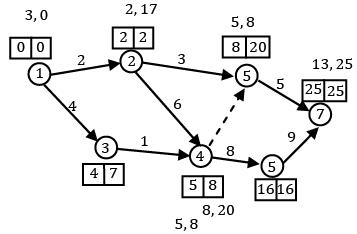Courses

# Test: CPM And PERT (Level - 2)

## 20 Questions MCQ Test Manufacturing and Industrial Engineering | Test: CPM And PERT (Level - 2)

Description
This mock test of Test: CPM And PERT (Level - 2) for Mechanical Engineering helps you for every Mechanical Engineering entrance exam. This contains 20 Multiple Choice Questions for Mechanical Engineering Test: CPM And PERT (Level - 2) (mcq) to study with solutions a complete question bank. The solved questions answers in this Test: CPM And PERT (Level - 2) quiz give you a good mix of easy questions and tough questions. Mechanical Engineering students definitely take this Test: CPM And PERT (Level - 2) exercise for a better result in the exam. You can find other Test: CPM And PERT (Level - 2) extra questions, long questions & short questions for Mechanical Engineering on EduRev as well by searching above.
QUESTION: 1

Solution:
QUESTION: 2

Solution:
QUESTION: 3

### Which one of the following statements is not correct?

Solution:

(A) PERT → Event oriented CPM → Activity oriented

(B) PERT → 3 time estimates are made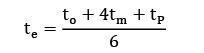to = optimistic time

tm = most likely time

tP = pessimistic time

CPM → only one time estimate

(C) In PERT slack is calculated, CPM floats calculated

(D) Both PERT and CPM are used for project management

QUESTION: 4

If the earliest starting time for an activity is 10 weeks, the latest finish time is 30 weeks and the duration time of the activity is 11 weeks, then the total float is equal to

Solution: T. F. = LSi − ESij − tij = 30 − 10 − 11 = 9

QUESTION: 5

The earliest occurrence time for event '1' is 8 weeks and the latest occurrence time for event' 1' is 26 weeks. The earliest occurrence time for event '2' is 32 weeks and the latest occurrence time for event '2' is 37 weeks.

If the activity time is 11 weeks, then the total float will be

Solution: Total float = 37 − 8 − 11 = 18 days

QUESTION: 6

Consider an activity having a duration time of Tij. E is the earliest occurrence time and L the latest occurrence time (see figure given).

Consider the following statements in this regard: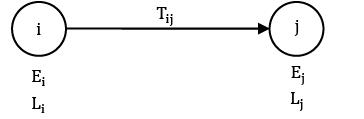1. Total float = Lj − Ei − Tij

2. Free float = Ej − Ei − Tij

3. Slack of the tail event = Lj − Ei

Of these statements

Solution:
QUESTION: 7

In a small engineering project, for an activity, the optimistic time is 2 minutes, the most likely time is 5 minutes and the pessimistic time is 8 minutes. What is the expected time of the activity?

Solution:

Expected time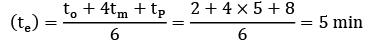QUESTION: 8

Consider the following project network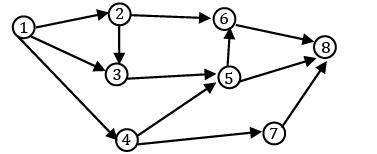The number of merge events are

Solution: Merge event: If two or more than two activities are having same end event, then that event is known as merge event Merge events: 3, 5, 6, 8

QUESTION: 9

For the network shown in the given figure, the expected completion time of the project is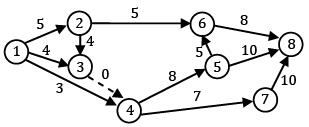Solution:

Paths are: 1-2-6-8 = 18

1-2-3-4-5-6-8 = 30

1-2-3-4-5-8 = 27

1-2-3-4-7-8 = 26

1-3-4-5-6-8 = 25

1-3-4-5-8 = 22

1-3-4-7-8 = 21

1-4-5-6-8 = 24

1-4-5-8 = 21

1-4-7-8 = 20

QUESTION: 10

The total float associated with the activity shown below is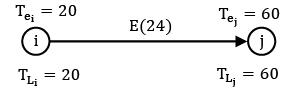Solution: Total float = LFT − (EST + Tij)

= 60 − (20 + 24)

= 16

QUESTION: 11

Consider the network. Activity times are given in number of days. The earliest expected occurrence time (TE) for event 50 is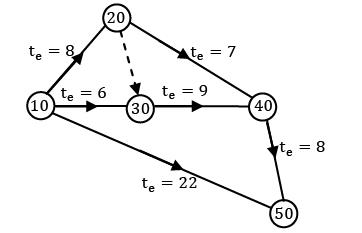Solution: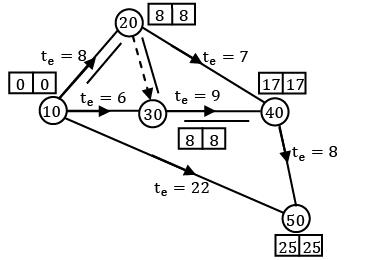Critical path is given by 10 − 20 − 30 − 40 − 50

∴The earliest expected occurrence time (TE) for the event is 25

QUESTION: 12

The variance (V1) for critical path a → b = 4 time units, b → c = 16 time units, c → d = 4 time units, d → e = 1 time unit. The standard deviation ‘d’ of the critical path a → e is

Solution: Standard Deviation = √4 + 16 + 4 + 1 = 5

QUESTION: 13

Consider an activity ‘A’ which is having the following values:

NC = Rs 100

NT = 3 days

CC = Rs 150

CT = 2 days

Find the cost of crashing activity A’ by single day

Solution: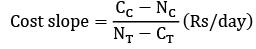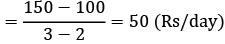*Answer can only contain numeric values
QUESTION: 14

A project consist of following activities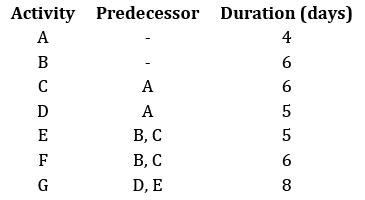Minimum time required to complete the project in (days) is __________

Solution: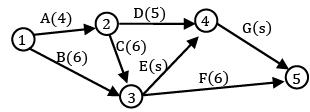A‐ D‐ G = 4 + 5 + 8 = 17

A‐C‐E‐G = 4 + 6 + 5 + 8 = 23

B‐ E‐ G = 6 + 5 + 8 = 19

B‐ F = 6 + 6 = 12

A‐ C‐ F = 4 + 6 + 6 = 16

QUESTION: 15

For the given project, to draw a network diagram how many dummy activities are required?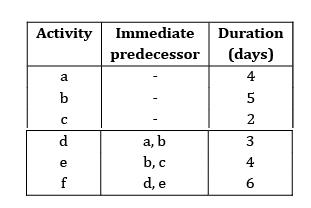Solution: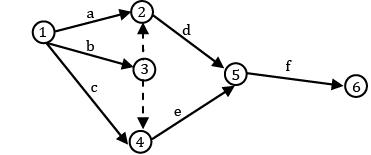No. of dummy activities required = 2

Question_type_5

QUESTION: 16

In the network shown below. The critical path is along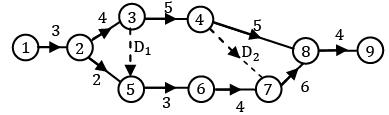Solution:

1-2-3-4-8-9 = 21

1-2-3-4-7-8-9 = 22

1-2-3-5-6-7-8-9 = 24

1-2-5-6-7-8-9 = 22

QUESTION: 17

Consider the network shown in figure. The three time estimates for activities are given along the arrows. Then the standard deviation of the project is

Ans. A

Solution: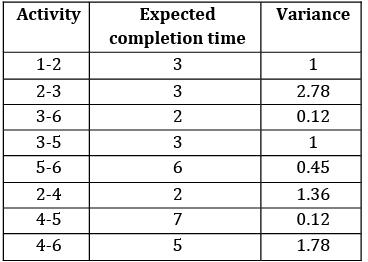Hence critical path is 1-2-3-4-5-6 Standard deviation is σ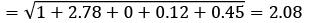*Answer can only contain numeric values
QUESTION: 18

Consider the following project find the critical path duration.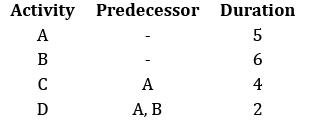Solution: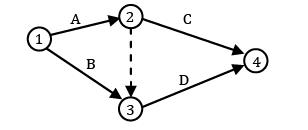A‐ C = 9

A‐ D = 7

B‐ D = 8

QUESTION: 19

The expected project completion time is found to be 34 days. The standard deviation of the critical path is 5 days. Find the probability of completing the project in 39 days.

Ans. B

Solution: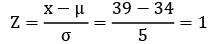P = 0.84

QUESTION: 20

For the project network shown below calculate latest finish time of node 4.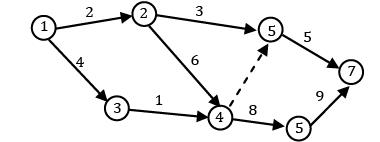Solution: